ISEE Middle Level Math : How to add fractions

Example Questions

Example Question #61 : How To Add Fractions

Which of the following is the sum of seven tenths and seventeen hundredths?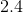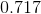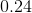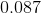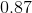Explanation:

Seven tenths is equal to 0.7; seventeen hundredths is equal to 0.17. Add them, rewriting 0.7 as 0.70: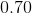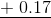Example Question #62 : How To Add Fractions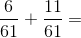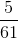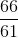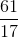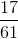Explanation:

Add the numerators and leave the denominators the same: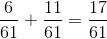Example Question #63 : How To Add Fractions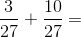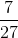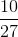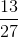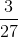Explanation:

To solve, add the numerators and leave the denominators the same: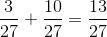Example Question #64 : How To Add Fractions

Find the sum.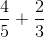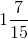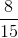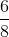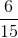Explanation:

When adding fractions, you must first find a common denominator.  You can find a common denominator by multiplying the numerator and denominator of each fraction by the denominator of the other. Once you have a common denominator, add the numerators and keep the denominator the same.Then simplify or convert to a mixed number if necessary.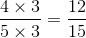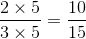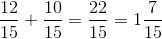Example Question #65 : How To Add Fractions

Find the sum.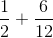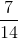Explanation:

When adding fractions, you must first find a common denominator.  Since the denominator in the second fraction (12) is a multiple of the denominator in the first fraction (2), you can keep the second fraction the same and multiply the numerator and denominator in the first fraction by the number you need to multiply by the first denominator to produce the second denominator.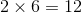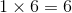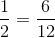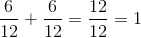Example Question #66 : How To Add Fractions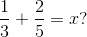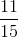Explanation:

To add fractions you must have a common denominator.  They share a multiple of.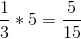and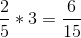.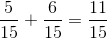.

Example Question #67 : How To Add Fractions

Find the sum: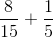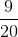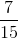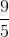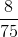Explanation:

To add fractions, you must have a common denominator.

Sinceis a multiple of, we can use.

We must multiple the one fraction byto get the denominator towhich makes the numerator.

With like denominators, you just add the numerators together which gives us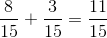.

Example Question #68 : How To Add Fractions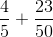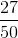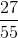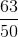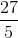Explanation:First, we need a common denominator. We can get one by multiplying "four-fifths" by "ten-tenths." Then, add the numerators to get the answer.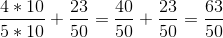Example Question #69 : How To Add Fractions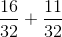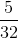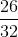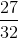Explanation:

To solve, add the numerators and keep the denominators the same: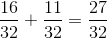Example Question #70 : How To Add Fractions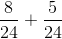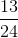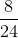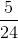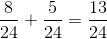Answer: